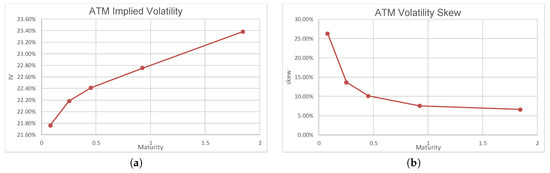Next Article in Journal
Some Nonlocal Operators in the First Heisenberg Group
Next Article in Special Issue
Option Pricing Models Driven by the Space-Time Fractional Diffusion: Series Representation and Applications
Previous Article in Journal
The Kernel of the Distributed Order Fractional Derivatives with an Application to Complex Materials
Open AccessArticle

# A Solution to the Time-Scale Fractional Puzzle in the Implied Volatility

byHideharu Funahashi 1,* andMasaaki Kijima 2
1
Mizuho Securities Co. Ltd., Tokyo 100-0004, Japan
2
Master of Finance Program, Tokyo Metropolitan University, Tokyo 100-0005, Japan
*
Author to whom correspondence should be addressed.
Fractal Fract 2017, 1(1), 14; https://doi.org/10.3390/fractalfract1010014
Received: 28 October 2017 / Revised: 22 November 2017 / Accepted: 22 November 2017 / Published: 25 November 2017
(This article belongs to the Special Issue Fractional Calculus in Economics and Finance)
In the option pricing literature, it is well known that (i) the decrease in the smile amplitude is much slower than the standard stochastic volatility models and (ii) the term structure of the at-the-money volatility skew is approximated by a power-law function with the exponent close to zero. These stylized facts cannot be captured by standard models, and while (i) has been explained by using a fractional volatility model with Hurst index $H > 1 / 2$ , (ii) is proven to be satisfied by a rough volatility model with $H < 1 / 2$ under a risk-neutral measure. This paper provides a solution to this fractional puzzle in the implied volatility. Namely, we construct a two-factor fractional volatility model and develop an approximation formula for European option prices. It is shown through numerical examples that our model can resolve the fractional puzzle, when the correlations between the underlying asset process and the factors of rough volatility and persistence belong to a certain range. More specifically, depending on the three correlation values, the implied volatility surface is classified into four types: (1) the roughness exists, but the persistence does not; (2) the persistence exists, but the roughness does not; (3) both the roughness and the persistence exist; and (4) neither the roughness nor the persistence exist. View Full-Text
Show FiguresFigure 1

MDPI and ACS Style

Funahashi, H.; Kijima, M. A Solution to the Time-Scale Fractional Puzzle in the Implied Volatility. Fractal Fract 2017, 1, 14.